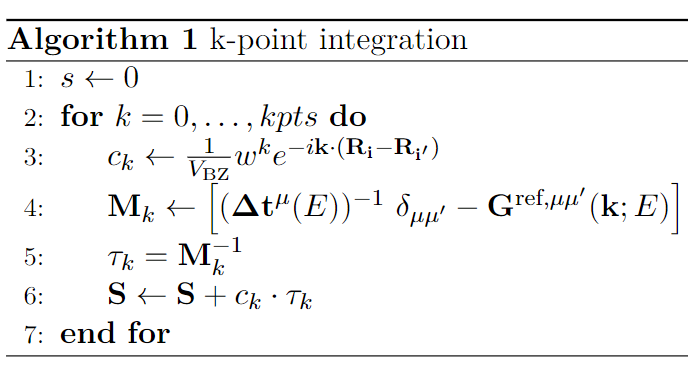# JuKKR kloop (original)

Version's name: JuKKR kloop (original) ; a version of the juKKR kloop program.
Patterns and behaviours: Recommended best-practices:

This version of the JuKKR-kloop kernel represents the original implementation of the k-point integration loop as found in the KKRhost code of the JuKKR code family. For simplicity it performs the k-point integration only for two selected energy points. These energy points are taken from a real simulation run of KKRhost on a 3D unit cell of a crystal lattice containing 4 gold atoms.

Algorithmically, the k-point integration loop looks as follows:The variables in the algorithm have the following types: | Type | Variable | | :——: | :—-: | | Scalar | $$c_k$$, $$V_{BZ}$$, $$w^k$$, $$e$$ | | Vector | $$\textbf{k}$$, $$\textbf{R}_i$$, $$\textbf{R}_{i'}$$ | | Matrix | $$\textbf{M}_k$$, $$\textbf{\Delta t}_k$$, $$\delta_{\mu \mu'}$$, $$\textbf{G}_k$$, $$\textbf{\tau}_k$$, $$\textbf{S}_k$$ |

First the exponential prefactor $$c_k$$ is constructed. The computation of the matrix $$M_k$$ involves an inverse Fourier transformation of $$G^{ref}$$ to get $$G^{ref, \mu\mu'}$$. Afterwards the inversion of $$M_k$$ is done by computing an LU decomposition using the LAPACK routine $$\texttt{zgetrf}$$.

In this version of the kernel the outer loop over all k-points is not parallelized. Note that in the full KKRhost application the k-points are distributed over multiple MPI ranks. So our kernel represents the computations that an individual MPI rank has to perform. For the LU decompositions in line 5 the threaded version of the Intel MKL is used to exploit OpenMP parallelism inside the MPI process.

This implementation leads to a sequence of very fine grained parallel regions. In terms of performance this causes a very bad scaling of the number of instructions per cycle (IPC) when increasing the number of OpenMP threads used. The reason is that the matrices $$M_k$$ are very small. In this context a typical size for these matrices is $$32 \times 32$$. Even for complex type matrices as in this case such a matrix easily fits into the L1-cache of a single core of current processors. If multiple OpenMP threads collaborate to compute the LU decomposition inside the Intel MKL call we probably see False-Sharing effects as the matrix probably resides in the cache of the CPU core that the master thread (= MPI process) runs on.

The following experiments have been registered: